The AUTOREG Procedure
 OUTEST= Data Set

The OUTEST= data set contains all the variables used in any MODEL statement. Each regressor variable contains the estimate for the corresponding regression parameter in the corresponding model. In addition, the OUTEST= data set contains the following variables:

_A_i

the ith order autoregressive parameter estimate. There are m such variables _A_1 through _A_m, where m is the value of the NLAG= option.

_AH_i

the ith order ARCH parameter estimate, if the GARCH= option is specified. There are q such variables _AH_1 through _AH_q, where q is the value of the Q= option. The variable _AH_0 contains the estimate of
.

_AHP_i

the estimate of the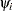parameter in the PGARCH model, if a PGARCH model is specified. There are q such variables _AHP_1 through _AHP_q, where q is the value of the Q= option.

_AHQ_i

the estimate of theparameter in the QGARCH model, if a QGARCH model is specified. There are q such variables _AHQ_1 through _AHQ_q, where q is the value of the Q= option.

_AHT_i

the estimate of theparameter in the TGARCH model, if a TGARCH model is specified. There are q such variables _AHT_1 through _AHT_q, where q is the value of the Q= option.

_DELTA_

the estimated mean parameter for the GARCH-M model if a GARCH-in-mean model is specified

_DEPVAR_

the name of the dependent variable

_GH_i

the ith order GARCH parameter estimate, if the GARCH= option is specified. There are p such variables _GH_1 through _GH_p, where p is the value of the P= option.

_HET_i

the ith heteroscedasticity model parameter specified by the HETERO statement

INTERCEPT

the intercept estimate. INTERCEPT contains a missing value for models for which the NOINT option is specified.

_METHOD_

the estimation method that is specified in the METHOD= option

_MODEL_

the label of the MODEL statement if one is given, or blank otherwise

_MSE_

the value of the mean square error for the model

_NAME_

the name of the row of covariance matrix for the parameter estimate, if the COVOUT option is specified

_LAMBDA_

the estimate of the power parameter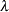in the PGARCH model, if a PGARCH model is specified.

_LIKLHD_

the log-likelihood value of the GARCH model

_SSE_

the value of the error sum of squares

_START_

the estimated start-up value for the conditional variance when GARCH= (STARTUP=ESTIMATE) option is specified

_STATUS_

This variable indicates the optimization status. _STATUS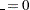indicates that there were no errors during the optimization and the algorithm converged. _STATUS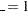indicates that the optimization could not improve the function value and means that the results should be interpreted with caution. _STATUS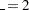indicates that the optimization failed due to the number of iterations exceeding either the maximum default or the specified number of iterations or the number of function calls allowed. _STATUS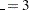indicates that an error occurred during the optimization process. For example, this error message is obtained when a function or its derivatives cannot be calculated at the initial values or during the iteration process, when an optimization step is outside of the feasible region or when active constraints are linearly dependent.

_STDERR_

standard error of the parameter estimate, if the COVOUT option is specified.

_TDFI_

the estimate of the inverted degrees of freedom for Student’s t distribution, if DIST=T is specified.

_THETA_

the estimate of the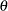parameter in the EGARCH model, if an EGARCH model is specified.

_TYPE_

OLS for observations containing parameter estimates, or COV for observations containing covariance matrix elements.

The OUTEST= data set contains one observation for each MODEL statement giving the parameter estimates for that model. If the COVOUT option is specified, the OUTEST= data set includes additional observations for each MODEL statement giving the rows of the covariance of parameter estimates matrix. For covariance observations, the value of the _TYPE_ variable is COV, and the _NAME_ variable identifies the parameter associated with that row of the covariance matrix.Previous Page | Next Page | Top of Page